# Definitions: Speaker Parameters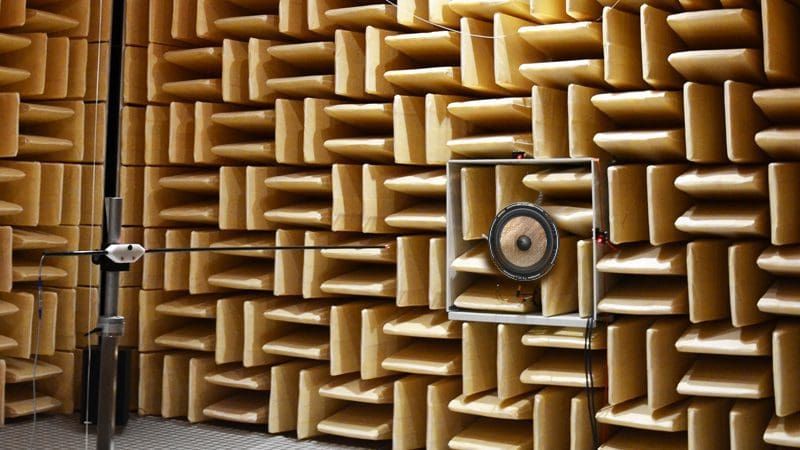The adage that someone could write a book about a subject certainly holds true when it comes to a discussion of loudspeakers and their parameters. In fact, there are dozens of great books already available about the subject. This article provides an overview of some of the most commonly discussed speaker parameters.

## What are Speaker Parameters?

Speaker parameters, often called Thiele/Small parameters, are a set of electromechanical measurements that can be used to define the low-frequency performance of a transducer. Using these parameters and a series of calculations, your installer can predict the performance of that speaker in an enclosure.

## What Can We Determine from these Parameters?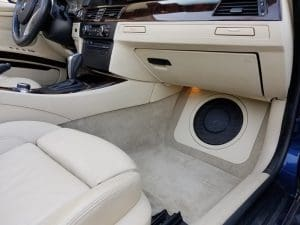T/S Parameters can be used to determine if speakers will work well in small enclosures.

Perhaps the most important set of calculations we can create is the output of the system. When we discuss the “system,” we are referring to the speaker itself and the enclosure in which we intend to install the speaker. Every speaker enclosure acts as a high-pass filter and reduces the low-frequency output of the driver. We gain physical power handling in return for this diminished output. Using a set of calculations, we can predict how much low-frequency information the system will produce.

Another important calculation is power handling. As mentioned, we need to control the movement of the speaker cone to prevent distortion and damage. We can predict how much the cone will move for a given amount of power in our test enclosure.

## Resonant Frequency of the Speaker – Fs

In terms of analyzing the moving parts of the speaker, we need to know the frequency at which the compliance (springiness) of the spider and the surround combine with the mass of the cone and dust cap to store the most energy. At this frequency, the system alternately stores and subsequently releases the most energy for a given voltage input. If you were to swing a weight on a string suspended from the ceiling, the natural frequency at which it oscillates back and force would be equal to the resonant frequency of a loudspeaker.

## Equivalent Compliance Volume – Vas

To understand how stiff the spider and the surround are, we compare them to an amount of air that would exert the same resistance to motion. Because air is easily compressed, a high Vas specification would represent a very softly suspended cone. Conversely, a speaker with a low Vas would have a very stiff suspension.

## Electrical Q of the Driver at Fs – Qes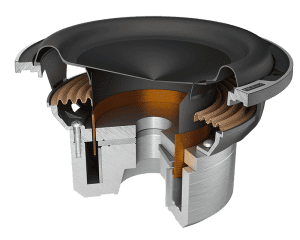Understanding the Q (Quality Factor) can be somewhat difficult because it is a dimension-less value. In essence, the Q factor describes the damping characteristic of a resonant system. A higher Q represents less energy loss relative to the total energy stored in a system. A pendulum suspended from a low-friction bearing will have a high Q. That same pendulum, submerged in water, will have a much lower Q. An important consideration is that high-Q systems have less damping and, therefore, vibrate longer. The Electrical Q specification describes how much damping the voice coil and magnet assembly invoke on the moving cone.

As the voice coil moves past the magnet, it produces an electrical current. This current reaches its peak value at the resonant frequency of the driver and counteracts the current being provided by the amplifier. The net result is a significant rise in impedance at the resonant frequency.

## Mechanical Q of the Driver at Fs – Qms

Just as the electrical characteristics of a speaker cause an opposition to cone motion, we have a similar effect from the mechanical properties of the speaker. Qms describes the mechanical losses resulting from the spider and the surround. A high Qms value describes lower mechanical losses, while a low Qms value describes higher losses.

## Total System Q at Fs – Qts

This unit-less measurement is a mathematical combination of the mechanical and electrical characteristics of the speaker. In simple terms, we calculate Qts by dividing the total stored energy of the speaker by the dissipated energy in the speaker at resonance.

## Compliance of the Driver Suspension – Cms

The Cms specification describes the stiffness of the driver suspension in meters per newton. A stiffer suspension will move less distance for a given amount of force applied to it.

## Effective Cone Area of the Driver – Sd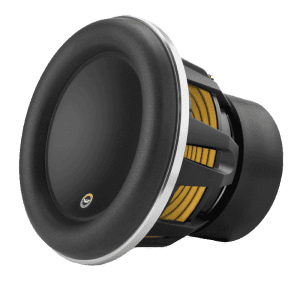This parameter describes the effective “size” of our speaker. We all realize that the cone will move air for us, but we also have to take into account the addition of the surround. It is commonly accepted that we can use a value of half the surround as contributing to the output of the driver.

## Mass of the Cone and Moving Parts – Mms

The Mms specification describes the mass of the speaker cone and part of the spider and surround. Unlike the Mmd specification, Mms includes the acoustic load caused by the air in contact with the cone. In most cases, the values are similar, but as the surface area of the cone increases, so too does the value of Mms, relative to Mmd.

## Maximum Excursion Level – Xmax

This parameter is frequently misinterpreted as being the defining factor in the distance a speaker cone can move. Early calculations used a formula that subtracted the height of the voice coil winding from the height of the magnetic gap, then divided by 2. This calculation describes how far the speaker can move before the winding comes out of the gap.

Subsequent investigation shows that non-linear behavior elsewhere in the driver design could have a larger influence on the motion limits of the cone. This suggests that Xmax should be the one-way excursion distance that represents a distortion level of 10%. This performance-oriented specification is far more indicative of the useful operating range of a driver, but is much harder to ascertain.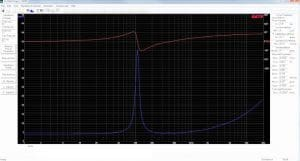In this article, we only describe the basic parameters that are commonly used in predicting the low-frequency performance of a loudspeaker. Other parameters, such as inductance, become more relevant at higher frequencies. Addition parameters such as Nominal Impedance (Znom), efficiency, sensitivity and the Efficiency Bandwidth Product (EBF) are derived through equations that use the specifications above.